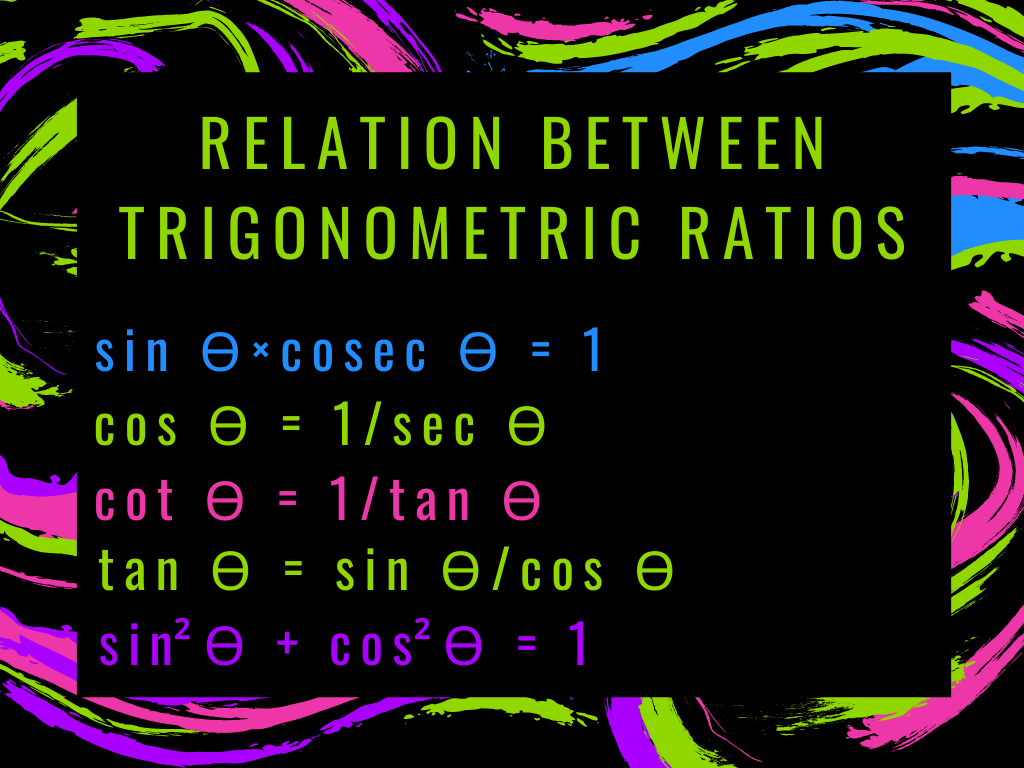# Relation Between Trigonometric Ratios Class 10th

## Introduction

We know that the reciprocal of sin ϴ, cos ϴ, and tan ϴ is cosec ϴ, sec ϴ, and cot ϴ respectively. Therefore, some formulae are here which show the Relation Between Trigonometric Ratios.

### Derivation of Formula and Explanation

If we assume △PQR,

sin ϴ = PQ/PR    and cosec ϴ = PR/PQ

sin ϴ⨯cosec ϴ = PQ/PR⨯PR/PQ

sin ϴ⨯cosec ϴ = 1

Similarly,

1) sin ϴ×cosec ϴ = 1  ⇒      sin ϴ = 1/cosec ϴ and       cosec ϴ = 1/sin ϴ

2) cos ϴ×sec ϴ = 1   ⇒      cos ϴ = 1/sec ϴ     and     sec ϴ = 1/cos ϴ

3) tan ϴ×cot ϴ = 1   ⇒    tan ϴ = 1/cot ϴ     and     cot ϴ = 1/ tan ϴ

4) tan ϴ = sin ϴ/cos ϴ

∵ sin ϴ = PQ/PR         and          cos ϴ = QR/PR

Now, sin ϴ/cos ϴ = PQ/PR ∕ QR/PR = PQ/PR×PR/QR = PQ/QR = Perpendicular/Base = tan ϴ

Similarly,

5) cot ϴ = cos ϴ/sin ϴ

### Trigonometric Identities

(1) sin2 ϴ + cos2 ϴ = 1

∵ sin ϴ = PQ/PR  and  cos ϴ = QR/PR [from the above figure]

LHS

sin2 ϴ + cos2 ϴ

(PQ/PR)2 + (QR/PR)2

PQ2/PR2 + QR2/PR2

PQ2 + QR2/PR2

PR2/PR2  = 1 = RHS           (by Pythagoras theorem PR2 = PQ2 + QR2)

(2) 1 + tan2 ϴ = sec2 ϴ

∵ tan ϴ = PQ/QR [from the above figure]

LHS

1 + tan2 ϴ

1 + (PQ/QR)2

1 + PQ2/QR2

QR2 + PQ2/QR2

PR2/QR2 = (PR/QR)2 = sec2 ϴ = RHS

Alternate Method –

We know that,  sin2 ϴ + cos2 ϴ = 1

Divide both sides by cos2 ϴ

sin2 ϴ/cos2 ϴ + cos2 ϴ/cos2 ϴ = 1/cos2 ϴ               (∵ tan ϴ = sin ϴ/cos ϴ)

tan2 ϴ + 1 = sec2 ϴ

(3) 1 + cot2 ϴ = cosec2 ϴ

∵ cot ϴ = QR/PQ [from the above figure]

LHS

1 + cot2 ϴ

1 + (QR/PQ)2

1 + QR2/PQ2

PQ2 + QR2/PQ2

PR2/PQ2 = (PR/PQ)2 = cosec2 ϴ = RHS

Alternate Method –

We know that,  sin2 ϴ + cos2 ϴ = 1

Divide both sides by sin2 ϴ

sin2 ϴ/sin2 ϴ + cos2 ϴ/sin2 ϴ = 1/sin2 ϴ            (∵ cot ϴ = cos ϴ/sin ϴ)

1 + cot2 ϴ  = cosec2 ϴ

Note – 1) (sin ϴ)2 = sin2 ϴ ≠ sin ϴ2

It means (sin ϴ)2 can be written as sin2 ϴ but cannot be written as sin ϴ2. The same applies to other trigonometric functions.

2) Trigonometric identities can be written as –

Example – If sin ϴ = 5/13 then find all the trigonometric functions using the relation between trigonometric ratios, where ϴ is an acute angle.

Solution – Here, sin ϴ = 5/13

We know that   sin2 ϴ + cos2 ϴ = 1

(5/13)2 + cos2 ϴ = 1

cos2 ϴ = 1 – (25/169)

cos ϴ = √(169-25)/169 = √(144/169) = 12/13

∵ sec ϴ = 1/cos ϴ

sec ϴ = 1 ∕ 12/13 = 13/12

∵ cosec ϴ = 1/sin ϴ = 1 ∕ 5/13 = 13/5

∵ 1 + cot2 ϴ = cosec2 ϴ

cot2 ϴ = cosec2 ϴ – 1

cot ϴ = √(13/5)2 – 1= √169/25 – 1 = √(169-25)/25 = √(144/25) = 12/5

∵ tan ϴ = 1/cot ϴ

tan ϴ = 1 ∕ 12/5 = 5/12

#### Examples Based on Trigonometric Identities

Example – 1) Prove that tan ϴ + cot ϴ = sec ϴ×cosec ϴ

Solution – tan ϴ + cot ϴ = sec ϴ×cosec ϴ

LHS         tan ϴ + cot ϴ

sin ϴ/cos ϴ + cos ϴ/sin ϴ

(sin ϴ⨯sin ϴ + cos ϴ⨯cos ϴ)/cos ϴ⨯sin ϴ

(sin2 ϴ + cos2 ϴ)/cos ϴ⨯sin ϴ {∵ sin2 ϴ + cos2 ϴ = 1}

(1/cos ϴ)⨯sin ϴ = sec ϴ × cosec ϴ = RHS

Example – 2) Prove that (1 + cot2 ϴ)(1 + cos ϴ)(1 – cos ϴ) = 1

Solution – (1 + cot2 ϴ)(1 + cos ϴ)(1 – cos ϴ) = 1

LHS          (1 + cot2 ϴ)(1 + cos ϴ)(1 – cos ϴ) {∵ 1 + cot2 ϴ = cosec2 ϴ}

(cosec2 ϴ)(1 – cos2ϴ)      {(a+b)(a-b) = a2 – b2}

cosec2 ϴ×sin2 ϴ {∵ 1 – cos2 ϴ = sin2 ϴ}

(1/sin2ϴ)×sin2ϴ               {cosec ϴ = 1/sinϴ}

1 = RHS

Relation Between Trigonometric Ratios Class 10th in Hindi

More About Relation Between Trigonometric Ratios

Rate this post

### 1 thought on “Relation Between Trigonometric Ratios Class 10th”

1.I dugg some of you post as I thought they were extremely helpful handy